Learn Tech Skills from Scratch @ Scaler EDGE# Dynamic Programming

## What is Dynamic Programming?

• Dynamic Programming (commonly referred to as DP) is an algorithmic technique for solving a problem by recursively breaking it down into simpler subproblems and using the fact that the optimal solution to the overall problem depends upon the optimal solution to it’s individual subproblems.
• The technique was developed by Richard Bellman in the 1950s.
• DP algorithm solves each subproblem just once and then remembers its answer, thereby avoiding re-computation of the answer for similar subproblem every time.
• It is the most powerful design technique for solving optimization related problems.
• It also gives us a life lesson - Make life less complex. There is no such thing as big problem in life. Even if it appears big, it can be solved by breaking into smaller problems and then solving each optimally.

## Applications

Before diving into DP, let us first understand where do we use DP.

The core concept of DP is to avoid repeated work by remembering partial results (results of subproblems). This is very critical in terms of boosting performance and speed of algorithm. Most of the problems in computer science and real world can be solved using DP technique.

• In real life scenarios, consider the example where I have to go from home to work everyday. For the first time, I can calculate the shortest path between home and work by considering all possible routes. But, it is not feasible to do the calculation every day. Hence, I will be memorizing that shortest path and will be following that route everyday. In computer science terms, Google Maps will be using DP algorithm to find the shortest paths between two points.

• Largest Common Subsequence (LCS) problem - Basis of data comparison problems and to identify plagiarism in the contents.

• Longest Increasing Subsequence problem - used in DNA Matching between two individuals. Generally, the DNAs are represented as strings and to form a match between DNAs of two individuals, the algorithm needs to find out the longest increasing sub sequence between them. In cases of DNA match, the longest common sub-string (LCS) is also found.

• Knapsack Problem You have a bag of limited capacity and you decide to go on a challenging trek. Due to the capacity restriction, you can only carry certain items in optimum quantity. How do you select the materials and its quantity in efficient manner so that you don’t miss out on important items? That’s where DP comes into aid.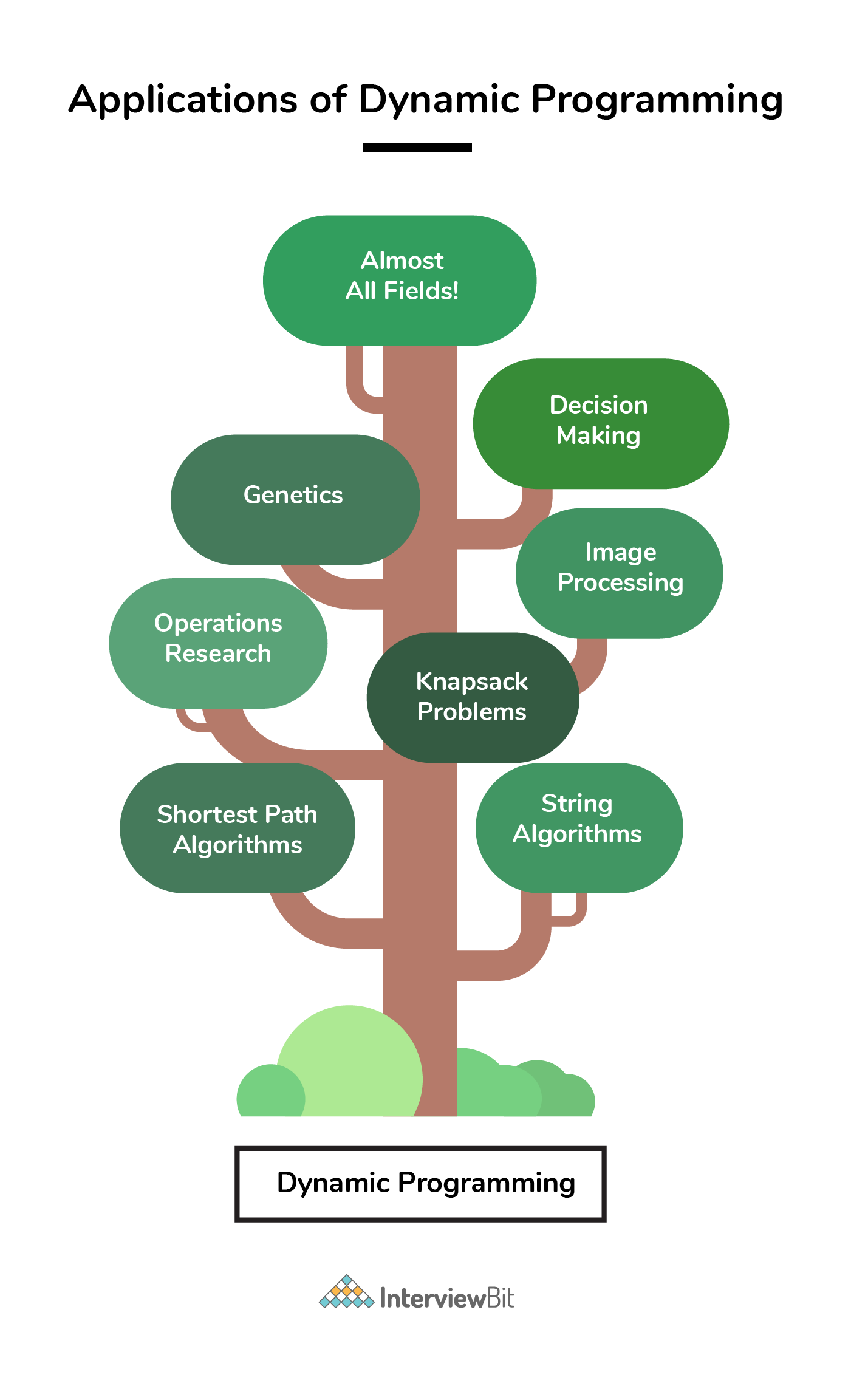• Apart from the above, DP has found its importance in various fields like Bioinformatics, Operations research, Decision Making, Image Processing, MATLAB, MS Word, MS Excel, Financial Optimisations, Genetics, XML indexing and querying and what not! Read More

### Important tutorials

1. Dynamic Programming ( Dp ) Introduction :
2. Dynamic Programming Examples :

## Dynamic Programming Problems

2d string dp
Greedy or dp
Problem Score Companies Time Status
Tushar's Birthday Bombs 200
72:34
Jump Game Array 225 40:51
Min Jumps Array 300 69:34
Matrix dp
Suffix / prefix dp
Problem Score Companies Time Status
Sub Matrices with sum Zero 200
67:10
Coin Sum Infinite 225
62:10
Best Time to Buy and Sell Stocks I 300 27:45
Max Product Subarray 300 62:58
Arrange II 350 66:44
Derived dp
Problem Score Companies Time Status
Chain of Pairs 200 27:07
Max Sum Without Adjacent Elements 225 56:47
Knapsack
Problem Score Companies Time Status
Tushar's Birthday Party 200 63:49
Flip Array 200
70:48
0-1 Knapsack 200 36:28
Equal Average Partition 350 76:03
Problem Score Companies Time Status
Best Time to Buy and Sell Stocks II 225 39:43
Dp optimized backtrack
Problem Score Companies Time Status
Word Break II 350 62:34
Tree dp
Problem Score Companies Time Status
Max Sum Path in Binary Tree 400 53:29
Multiply dp
Problem Score Companies Time Status
Unique Binary Search Trees II 400 34:21
Count Permutations of BST 400
67:14
Breaking words
Problem Score Companies Time Status
Word Break 400 63:14
Palindrome Partitioning II 400 57:58

Problem Score Companies Time Status
Tiling With Dominoes 200 51:15
Increasing Path in Matrix 200
33:21
Egg Drop Problem! 450 50:48
Paint House! 200
25:11
Minimum Difference Subsets! 200 34:58
Subset Sum Problem! 200 29:52
Merge elements 300 53:35
Max edge queries! 200 57:15

## Characteristics of Dynamic Programming

Before moving on to approaches to solve a DP problem, let us have a look at the characteristics of a problem upon which we can apply the DP technique.

We can apply DP technique to those problems that exhibit the below 2 characteristics:

### 1. Optimal Substructures

• Any problem is said to be having optimal substructure property if its overall optimal solution can be evaluated from the optimal solutions of its subproblems.
• Consider the example of Fibonacci Numbers.
• We know that a nth Fibonacci number (Fib(n)) is nothing but sum of previous 2 fibonacci numbers, i.e: `Fib(n) = Fib(n-1) + Fib(n-2)`.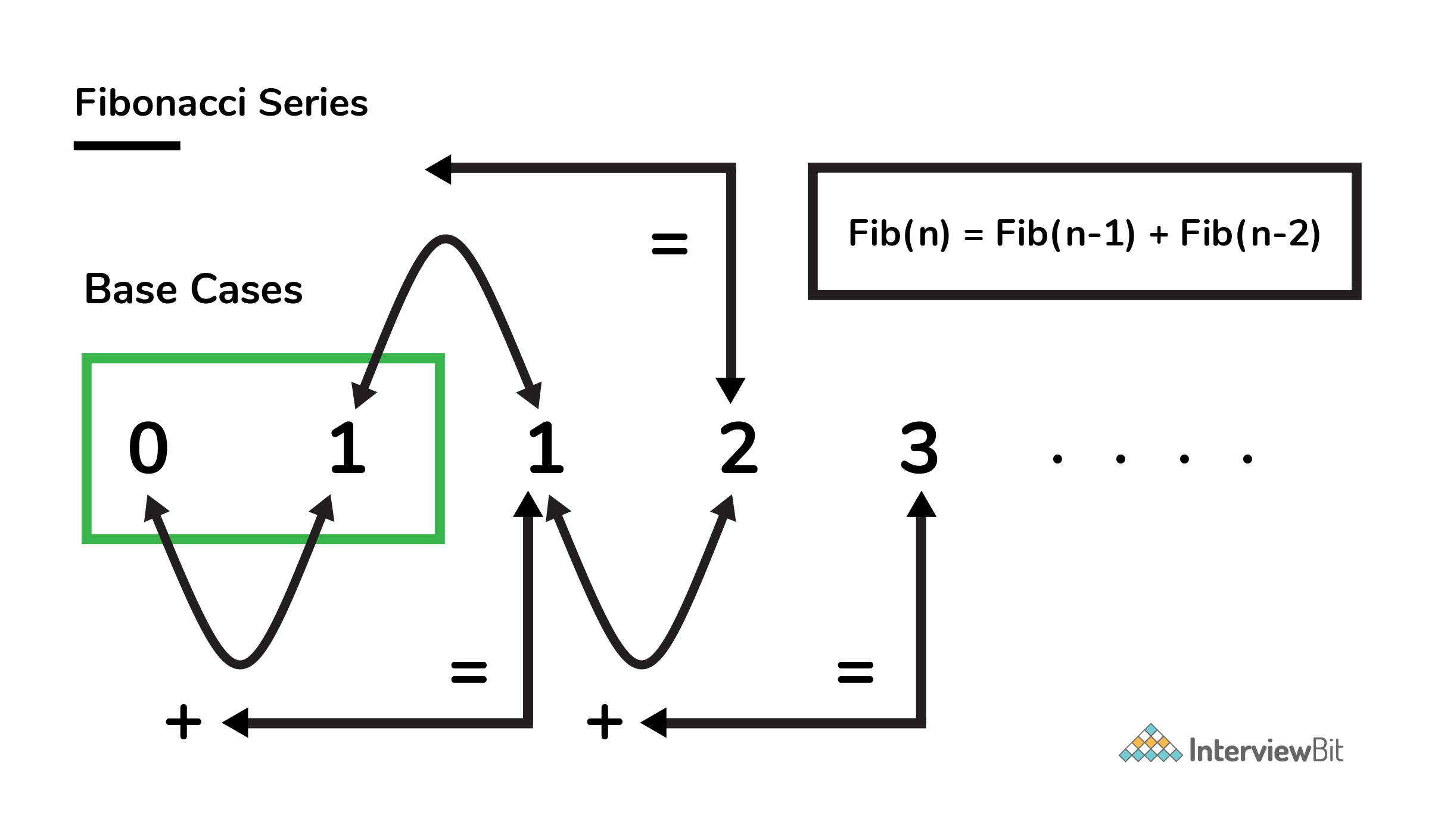• From the above equation, we can clearly deduce that a problem of size ‘n’ has been reduced to subproblems of size ‘n-1’ and ‘n-2’.

• Hence, we can say that Fibonacci numbers have the optimal substructure property.

### 2. Overlapping Subproblems

• Subproblems are basically the smaller versions of an original problem. Any problem is said to have overlapping subproblems if calculating its solution involves solving the same subproblem multiple times.
• Let us take the example of finding nth Fibonacci number. Consider evaluating Fib(5). As shown in the breakdown of steps shown in the image below, we can see that Fib(5) is calculated by taking sum of Fib(4) and Fib(3) and Fib(4) is calculated by taking sum of Fib(3) and Fib(2) and so on. Clearly, we can see that the Fib(3), Fib(2), Fib(1) and Fib(0) has been repeatedly evaluated. These are nothing but the overlapping subproblems.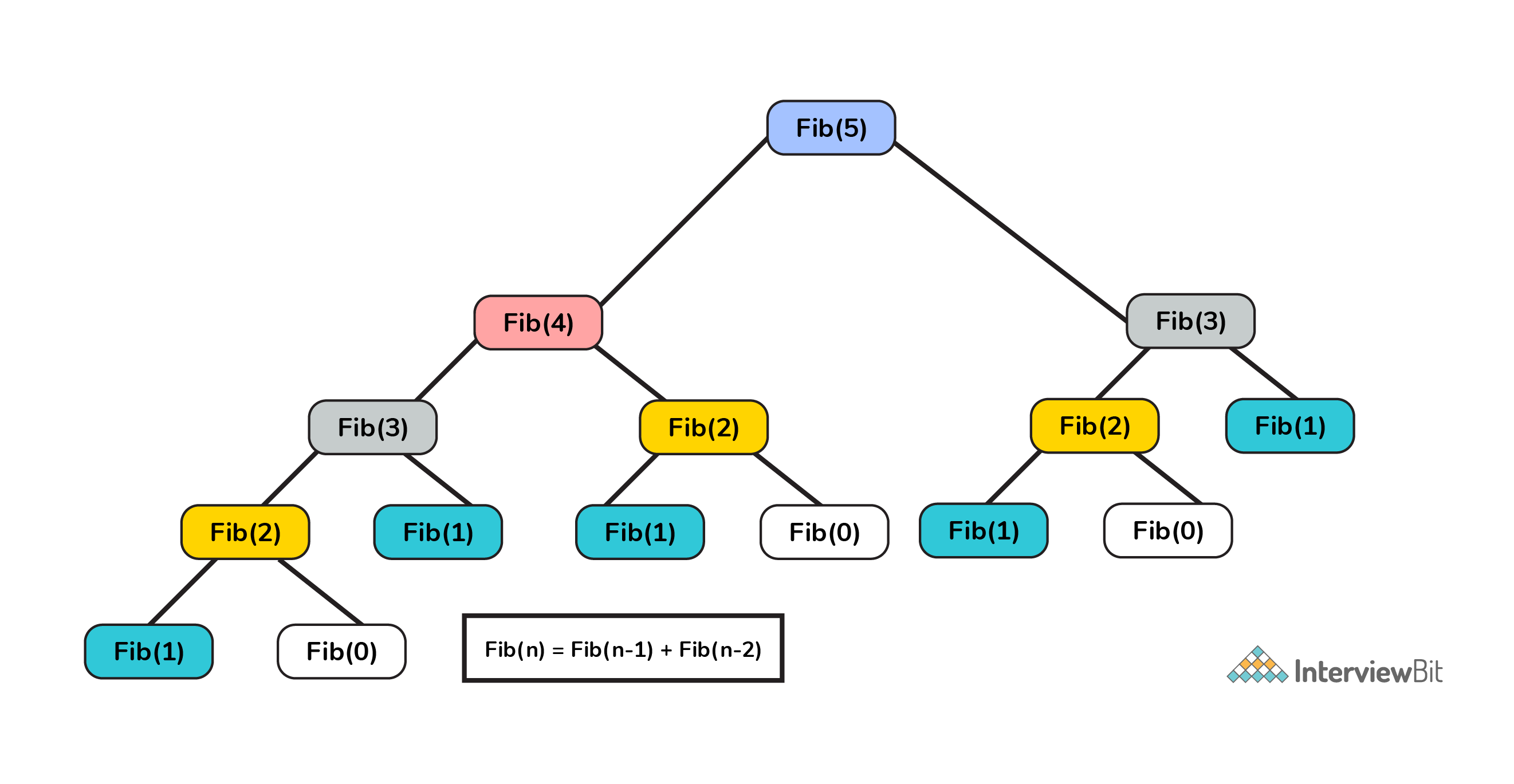``Note: It is important for a problem to have BOTH the above specified characteristics in order to be eligible to be solved using DP technique.``

## Dynamic Programming Methods

We shall continue with the example of finding the nth Fibonacci number in order to understand the DP methods available. We have the following two methods in DP technique. We can use any one of these techniques to solve a problem in optimised manner.

### Top Down Approach (Memoization)

• Top Down Approach is the method where we solve a bigger problem by recursively finding the solution to smaller sub-problems.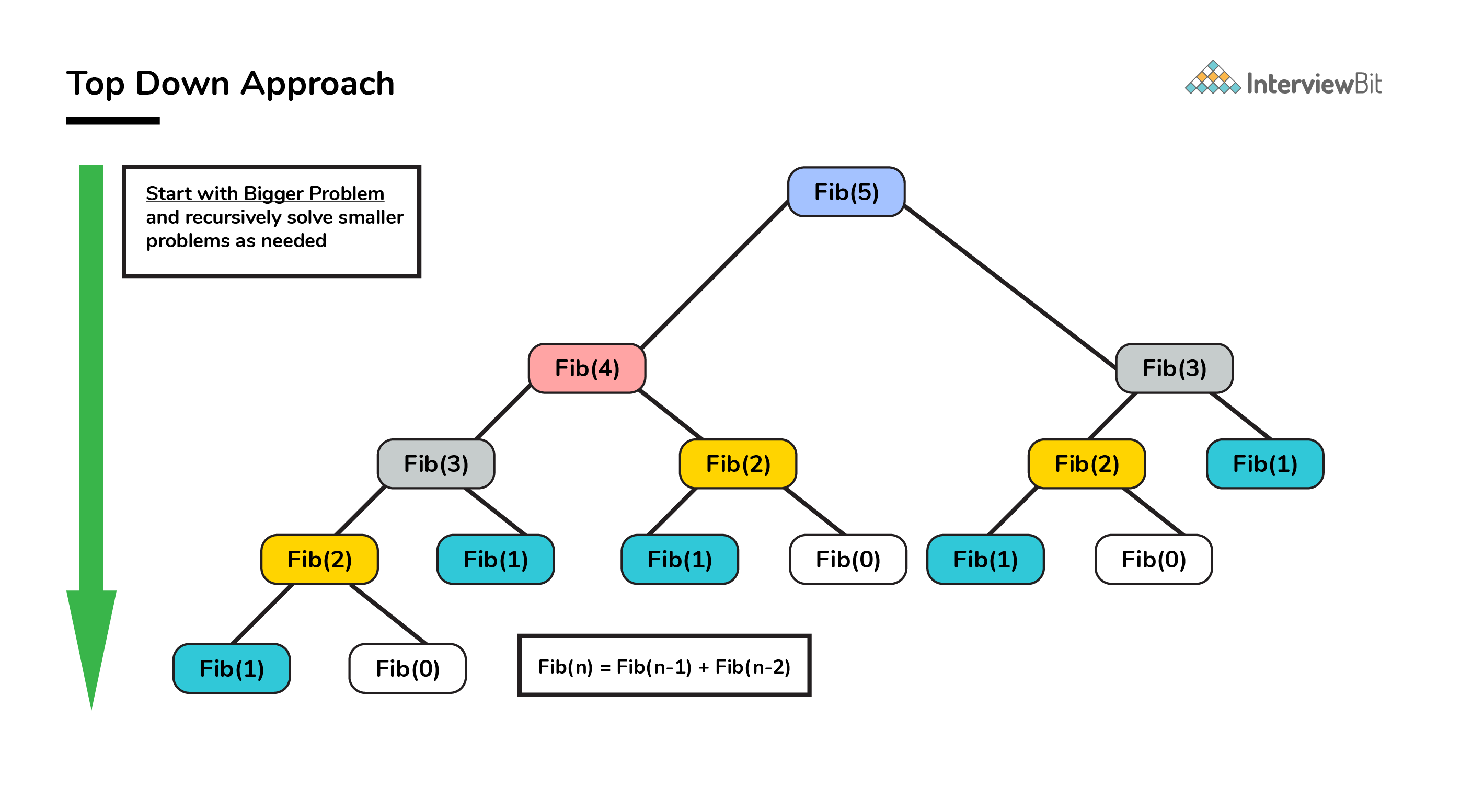• Whenever we solve a smaller subproblem, we remember (cache) its result so that we don’t solve it repeatedly if it’s called many times. Instead of solving repeatedly, we can just return the cached result.

• This method of remembering the solutions of already solved subproblems is called Memoization.

#### Pseudo Code and Analysis

Without Memoization

1. Think of a recursive approach to solving the problem. This part is simple. We already know `Fib(n) = Fib(n - 1) + Fib(n - 2)`.

2. Write a recursive code for the approach you just thought of.

``````/**
* Pseudo code for finding Fib(n) without memoization
* @Parameters: n : nth Fibonacci term needed
*
*/

int Fib(int n) {
if (n <= 1) return n;           //Fib(0)=0; Fib(1)=1
return Fib(n - 1) + Fib(n - 2);
}
``````
3. The time complexity of the above approach based on careful analysis on the property of recursion shows that it is essentially exponential in terms of n because some terms are evaluated again and again.

With Memoization

1. Save the results you get for every function run so that if `Fib(n)` is called again, you do not recompute the whole thing.
2. Instead of computing again and again, we save the value somewhere. This process of remembering the values of already run subproblem is called memoization.
3. Lets declare a global variable `memo` then.
``````    /**
* Pseudo code for finding Fib(n) with memoization
* @Parameters: n : nth Fibonacci term needed
*
*/
int memo = {0};
int Fib(int n) {
if (n <= 1) return n;

// If we have processed this function before,
// return the result from the last time.
if (memo[n] != 0) return memo[n];

// Otherwise calculate the result and remember it.
memo[n] = Fib(n - 1) + Fib(n - 2);
return memo[n];
}
``````
4. Let us now analyze the space and time complexity of this solution. We can try to improve this further if at all it is possible.
• Lets look at the space complexity first.
• We use an array of size n for remembering the results of subproblems. This contributes to a space complexity of `O(n)`.
• Since we are using recursion to solve this, we also end up using stack memory as part of recursion overhead which is also `O(n)`. So, overall space complexity is `O(n) + O(n) = 2 O(n) = O(n)`.
• Lets now look at the time complexity.
• Lets look at `Fib(n)`.

• When `Fib(n - 1)` is called, it makes a call to `Fib(n - 2)`. So when the call comes back to the original call from `Fib(n)``Fib(n-2)` would already be calculated. Hence the call to `Fib(n - 2)` will be O(1).

• Hence,

``````    T(n) = T(n - 1) + c where c is a constant.
= T(n - 2) + 2c
= T(n - 3) + 3c
= T(n - k) + kc
= T(0) + n * c = 1 + n * c = O(n)
``````

Thanks to Dynamic Programming, we have successfully reduced a exponential problem to a linear problem.

#### Implementation

``````/* C Program to find Nth Fibonacci Number using Memoization */

#include<stdio.h>

int Fibonacci_Series(int);
int memo = {0};
int main()
{
int N, FibN;

printf("\n Enter the Number to find Nth Fibonacci Number :  ");
scanf("%d", &N);

FibN = Fibonacci_Series(N);

printf("\n Fibonacci Number = %d", FibN);
return 0;
}

int Fibonacci_Series(int N)
{
if ( N == 0 )
return 0;
else if ( N == 1 )
return 1;

if (memo[n] != 0) return memo[n];
else{
memo[n]=Fibonacci_Series(N - 1) + Fibonacci_Series(N - 2)
return memo[n];
}
}``````
``````/* C++ Program to find Nth Fibonacci Number using Memoization */
#include <bits/stdc++.h>
using namespace std;
#define NIL -1

int memo;

/* Function to initialize NIL values in memo */
void initializeMemo()
{
int i;
for (i = 0; i < 100; i++)
memo[i] = NIL;
}

int fib(int n)
{
if (memo[n] == NIL)
{
if (n <= 1)
memo[n] = n;
else
memo[n] = fib(n - 1) + fib(n - 2);
}
return memo[n];
}

// Main Driver code
int main ()
{
int n = 10;
initializeMemo();
cout << "Fibonacci number : " << fib(n);
return 0;
}``````
``````/* Java Program to find Nth Fibonacci Number using Memoization */
public class Fibonacci
{
final int NIL = -1;

int memo[] = new int;

/* Function to initialize NIL values in memo */
void initializeMemo()
{
for (int i = 0; i < 100; i++)
memo[i] = NIL;
}

int fib(int n)
{
if (memo[n] == NIL)
{
if (n <= 1)
memo[n] = n;
else
memo[n] = fib(n-1) + fib(n-2);
}
return memo[n];
}

//Main Driver class
public static void main(String[] args)
{
Fibonacci fibonacci = new Fibonacci();
int n = 10;
fibonacci.initializeMemo();
System.out.println("Fibonacci number : " + fibonacci.fib(n));
}

}``````
``````# Python Program to find Nth Fibonacci Number using Memoization
def fib(n, memo):
# Base case
if n == 0 or n == 1 :
memo[n] = n

# If memo[n] is not evaluated before then calculate it
if memo[n] is None:
memo[n] = fib(n-1 , memo)  + fib(n-2 , memo)

# return the value corresponding value of n
return memo[n]

# Driver program
def main():
n = 10
# Declaration of memo
memo = [None]*(101)

# Calculate and display result
print("Fibonacci Number is " + str(fib(n, lookup)))

if __name__=="__main__":
main() ``````

### Bottom Up Approach (Tabulation)

• As the name indicates, bottom up is the opposite of the top-down approach which avoids recursion.

• Here, we solve the problem “bottom-up” way i.e. by solving all the related subproblems first. This is typically done by populating into an n-dimensional table.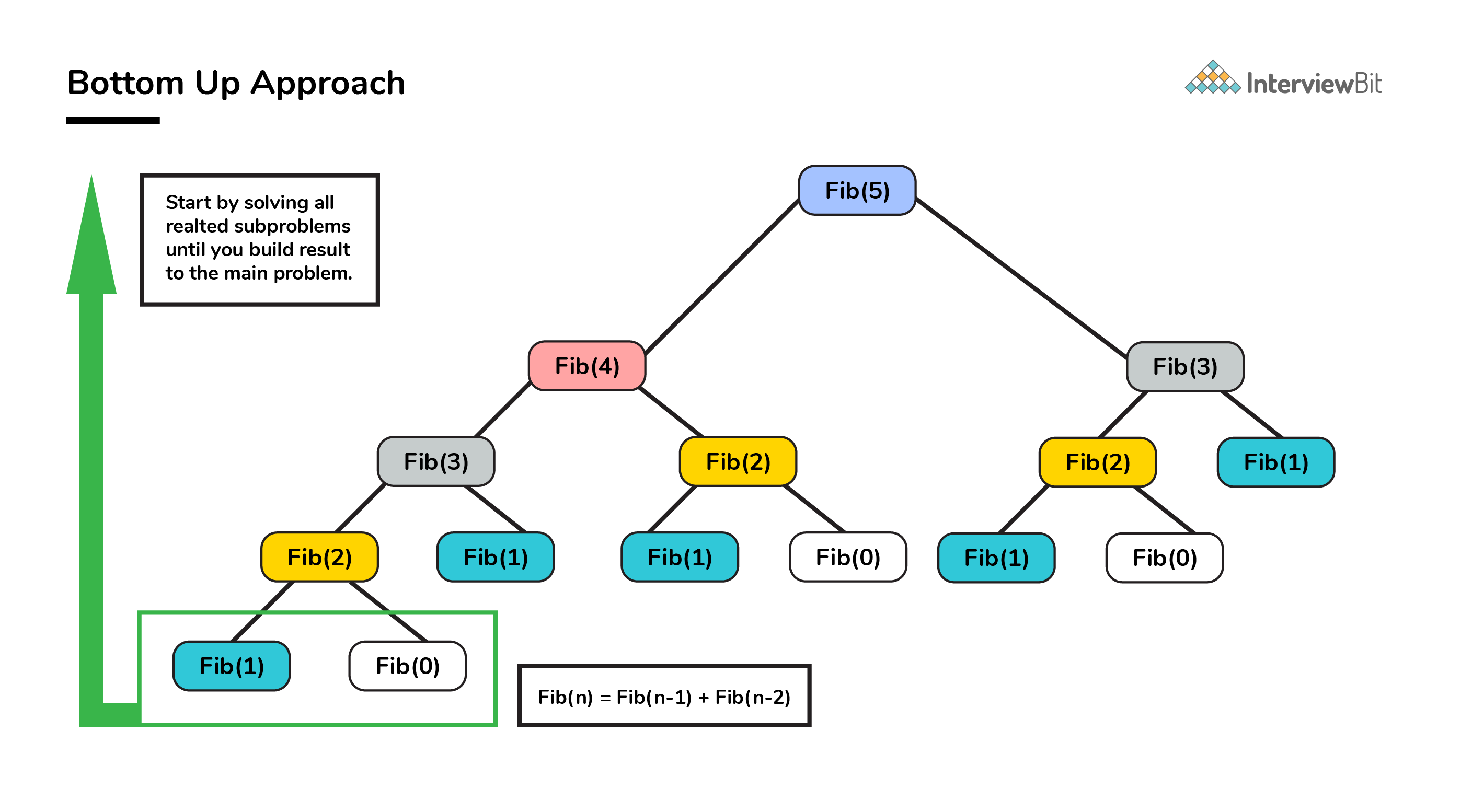• Depending on the results in the table, the solution to the original problem is then computed. This approach is therefore called as “Tabulation”.

#### Pseudo Code and Analysis

1. We already know `Fib(n) = Fib(n - 1) + Fib(n - 2)`.
2. Based on the above relation, we calculate the results of smaller subproblems first and then build the table.
``````/**
* Pseudo code for finding Fib(n) using tabulation
* @Parameters: n : nth Fibonacci term needed
* local variable dp[] table built to store results of smaller subproblems
*/

int Fib(int n) {
if (n==0) return 0;
int dp[] = new int[n+1];

//define base cases
dp = 0;
dp = 1;

//Iteratively compute the results and store
for(int i=2; i<=n; i++)
dp[i] = dp[i-1] + dp[i-2];

//return the value corresponding to nth term
return dp[n];
}
``````
1. Analyze the space and time requirements
• Lets look at the space complexity first.
• In this case too, we use an array of size n for remembering the results which contributes to a space complexity of `O(n)`.
• We can further reduce the space complexity from `O(N)` to `O(1)` by just using 2 variables. This is left as an assignment to the reader.
• Lets now look at the time complexity.
• Lets look at `Fib(n)`.
• Here, we solve each subproblem only once in iterative manner. So the time complexity of the algorithm is also O(N).
• Again thanks to DP, we arrived at solution in linear time complexity.

#### Implementation

``````/* C Program to find Nth Fibonacci Number using Tabulation */

#include<stdio.h>
int fib(int n)
{
int dp[n+1];
int i;
//base cases
dp = 0;   dp = 1;

//calculating and storing the values
for (i = 2; i <= n; i++)
dp[i] = dp[i-1] + dp[i-2];

return dp[n];
}

int main ()
{
int n = 10;
printf("Fibonacci number : %d ", fib(n));
return 0;
}``````
``````/* C++ Program to find Nth Fibonacci Number using Tabulation */
#include <bits/stdc++.h>
using namespace std;

int fib(int n)
{
int dp[n+1];
int i;

//base cases
dp = 0;   dp = 1;

//calculating and storing the values
for (i = 2; i <= n; i++)
dp[i] = dp[i-1] + dp[i-2];

return dp[n];
}

// Main Driver code
int main ()
{
int n = 10;
cout << "Fibonacci number : " << fib(n);
return 0;
}  ``````
``````/* Java Program to find Nth Fibonacci Number using Tabulation */
public class Fibonacci
{
int fib(int n){
int dp[] = new int[n+1];
//base cases
dp = 0;
dp = 1;

//calculating and storing the values
for (int i = 2; i <= n; i++)
dp[i] = dp[i-1] + dp[i-2];
return dp[n];
}

public static void main(String[] args)
{
Fibonacci fibonacci = new Fibonacci();
int n = 10;
System.out.println("Fibonacci number : " + fibonacci.fib(n));
}

} ``````
``````# Python Program to find Nth Fibonacci Number using Tabulation
def fib(n):

# dp array declaration
dp = *(n+1)

# base case
dp = 1

# calculating and storing the values
for i in xrange(2 , n+1):
dp[i] = dp[i-1] + dp[i-2]
return dp[n]

# Driver program
def main():
n = 10
print("Fibonacci number : " + str(fib(n)))

if __name__=="__main__":
main() ``````

## FAQs

• Why is dynamic programming named “dynamic”?

• According to Richard Bellman’s autobiography “Eye of the Hurricane: An Autobiography (1984)”, the word “dynamic” was chosen by him to mainly capture the time-varying aspect of the problems.
• How to recognize a problem that can be solved using Dynamic Programming?

• DP is mainly an optimization technique. It is a method for solving problems by breaking them down into simpler subproblems, solving and storing results of each subproblem just once. If the same subproblem occurs again, we look up for the previously stored solution.
• Hence, to recognize a problem whether it can be solved using DP, ask yourself whether the given problem solution can be expressed as a function of solutions to similar smaller subproblems.
• How to solve dynamic programming problems?

• The concept of dynamic programming is very simple. If we have solved a problem with the given input, then we save the result for future reference, so as to avoid recomputing again.

• If a given problem can be broken up in to smaller subproblems and these smaller subproblems can be in turn broken down in to even more smaller ones, and in this process, if we observe some subproblems which are already solved, then this is a big hint for us to use DP.
• In case we are not storing the results, then we are bound to perform computations unnecessarily which goes against the principle of dynamic programming.Image Source: Google
• We need to know that the optimal solutions to each subproblem contribute to the optimal solution of the overall given problem.

• We can follow the below steps as a guideline for coming up with a DP solution:

``````Step 1. Think of a recursive approach to solving the problem which essentially expresses a problem, say P(X), in terms of smaller subproblem, say P(Y) or an expression involving multiple smaller subproblems, say P(Yi). Here we expect Yi < X which could mean one of the following:
a. If X is an integer, then it could mean Yi "less than" X arithmetically.
b. If X is a string, it could mean that Yi is a substring of X.
c. If X is an array, it could mean Yi is a subarray of X, and so forth.

Step 2. Once you have a approach, write a recursive code for that. Consider your recursive code function definition to be as below :
solve(K1, K2, K3 ... )
Step 3. Keep track of the results of each function by saving them after every function call so that if the same function solve(K1, K2, K3 ... ) is called again, we need not compute again.

Step 4. Once we are done, analyze the space and time complexities of the solution developed, and try to improve them if possible.

``````

And that’s how a DP problem is solved.

• How is top down approach (memoization) different than bottom up approach (tabulation)?

Parameters Memoization Tabulation
State definition State definition can be thought of easily. Complicated to identify what a state should represent
Ease of code Less complicated and easy to code. Complications increase when lots of other conditions arise.
Speed of execution Slower due to recursive calls and return statements Faster as state values are accessed directly from table.
Space The cache entries are filled on demand during memoization. All entries starting from the first one needs to be filled by default.
• Where is dynamic programming used?

• Dynamic programming is used in the cases where we solve problems by dividing them into similar suproblems and then solving and storing their results so that results are re-used later.
• Used in the cases where optimization is needed.
• Please refer to Application section above.
• What are the characteristics of dynamic programming?

• Every DP problem should have optimal substructure and overlapping subproblems.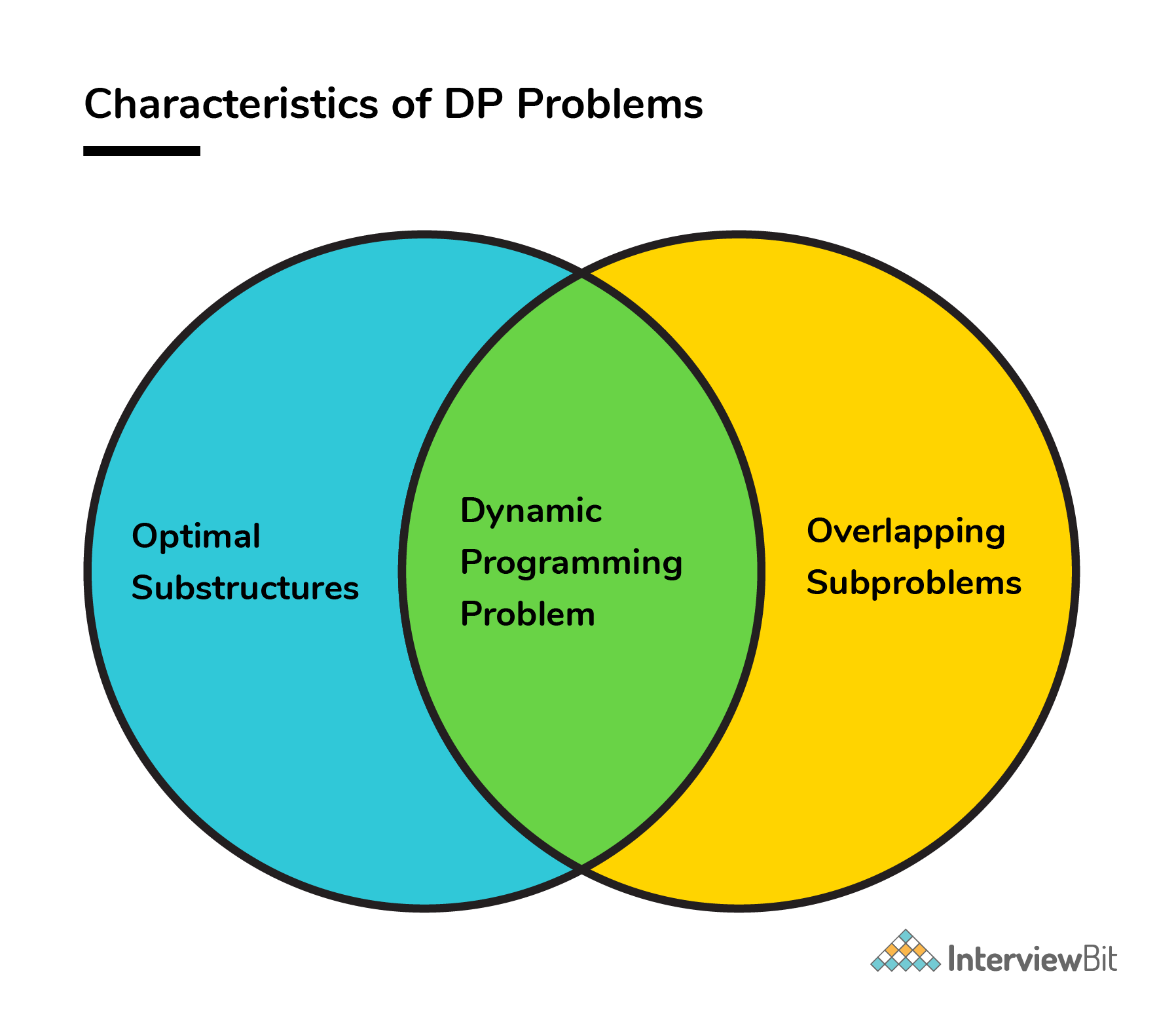• Please refer to Characteristics of Dynamic Programming section above.

• What are the applications of dynamic programming?

• DP is almost used everywhere which requires guaranteed optimal solution. It is heavily used in routing, graph problems, computer vision, computer networks, AI, machine learning etc.
• Please refer to Application section above.
• How is dynamic programming different from greedy approach?

Parameters Dynamic Programming Greedy Approach
Optimality There is guaranteed optimal solution as DP considers all possible cases and then choose the best among them. Provides no guarantee of getting optimum approach.
Memory DP requires a table or cache for remembering and this increases it’s memory complexity. More memory efficient as it never looks back or revises its previous choices.
Time complexity DP is generally slower due to considering all possible cases and then choosing the best among them. Generally faster.
Feasibility Decision at each step is made after evaluating current problem and solution to previously solved subproblem to calculate optimal solution. Choice is made which seems best at the moment in the hope of getting global optimal solution.
• How is dynamic programming different from divide and conquer approach?

• Divide and Conquer algorithm works by dividing a problem into subproblems, conquer by solving each subproblem recursively and then combine these solutions to get solution of the main problem.
• Whereas DP is a optimization technique for solving problems in an optimised manner by dividing problem into smaller subproblems and then evaluating and storing their results and constructing an optimal solution for main problem from computed information.
• The most important difference in Divide and Conquer strategy is that the subproblems are independent of each other. When a problem is divided into subproblems, they do not overlap which is why each subproblem is to be solved only once.
• Whereas in DP, a subproblem solved as part of a bigger problem may be required to be solved again as part of another subproblem (concept of overlapping subproblem), so the results of a subproblem is solved and stored so that the next time it is encountered, the result is simply fetched and returned.